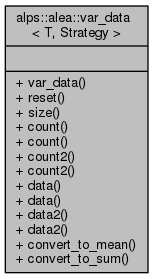ALPSCore reference
alps::alea::var_data< T, Strategy > Class Template Reference

#include <convert.hpp>

Inheritance diagram for alps::alea::var_data< T, Strategy >:Collaboration diagram for alps::alea::var_data< T, Strategy >:## Public Types

typedef bind< Strategy, T >::value_type value_type

typedef bind< Strategy, T >::var_type var_type

## Public Member Functions

var_data (size_t size)

void reset ()

size_t size () const

size_t count () const

size_t & count ()

double count2 () const

double & count2 ()

const column< value_type > & data () const

column< value_type > & data ()

const column< var_type > & data2 () const

column< var_type > & data2 ()

void convert_to_mean ()

void convert_to_sum ()

## Friends

class var_acc< T, Strategy >

class var_result< T, Strategy >

void serialize (serializer &, const std::string &, const var_result< T, Strategy > &)

void deserialize (deserializer &, const std::string &, var_result< T, Strategy > &)

## Detailed Description

### template<typename T, typename Strategy = circular_var> class alps::alea::var_data< T, Strategy >

Data for variance accumulation.

As with mean_acc, this class is basically a "union"-like structure, which for a data series (X, ... X[count_-1]) either represents the sum of X[i] and the sum of X[i]*X[i] (sum state) or the sample mean and sample variance of X (mean state).

Definition at line 19 of file convert.hpp.

## Member Typedef Documentation

template<typename T, typename Strategy = circular_var>
 typedef bind::value_type alps::alea::var_data< T, Strategy >::value_type

Definition at line 55 of file variance.hpp.

template<typename T, typename Strategy = circular_var>
 typedef bind::var_type alps::alea::var_data< T, Strategy >::var_type

Definition at line 56 of file variance.hpp.

## Constructor & Destructor Documentation

template<typename T , typename Str >
 alps::alea::var_data< T, Str >::var_data ( size_t size )

Definition at line 16 of file variance.cpp.

## Member Function Documentation

template<typename T , typename Str >
 void alps::alea::var_data< T, Str >::convert_to_mean ( )

Definition at line 33 of file variance.cpp.

template<typename T , typename Str >
 void alps::alea::var_data< T, Str >::convert_to_sum ( )

Definition at line 52 of file variance.cpp.

template<typename T, typename Strategy = circular_var>
 size_t alps::alea::var_data< T, Strategy >::count ( ) const
inline

Returns sample size, i.e., number of accumulated data points

Definition at line 68 of file variance.hpp.

template<typename T, typename Strategy = circular_var>
 size_t& alps::alea::var_data< T, Strategy >::count ( )
inline

Returns sample size, i.e., number of accumulated data points

Definition at line 71 of file variance.hpp.

template<typename T, typename Strategy = circular_var>
 double alps::alea::var_data< T, Strategy >::count2 ( ) const
inline

Returns sum of squared weights

Definition at line 74 of file variance.hpp.

template<typename T, typename Strategy = circular_var>
 double& alps::alea::var_data< T, Strategy >::count2 ( )
inline

Returns sum of squared weights

Definition at line 77 of file variance.hpp.

template<typename T, typename Strategy = circular_var>
 const column& alps::alea::var_data< T, Strategy >::data ( ) const
inline

Definition at line 79 of file variance.hpp.

template<typename T, typename Strategy = circular_var>
 column& alps::alea::var_data< T, Strategy >::data ( )
inline

Definition at line 81 of file variance.hpp.

template<typename T, typename Strategy = circular_var>
 const column& alps::alea::var_data< T, Strategy >::data2 ( ) const
inline

Definition at line 83 of file variance.hpp.

template<typename T, typename Strategy = circular_var>
 column& alps::alea::var_data< T, Strategy >::data2 ( )
inline

Definition at line 85 of file variance.hpp.

template<typename T , typename Str >
 void alps::alea::var_data< T, Str >::reset ( )

Re-allocate and thus clear all accumulated data

Definition at line 24 of file variance.cpp.

template<typename T, typename Strategy = circular_var>
 size_t alps::alea::var_data< T, Strategy >::size ( ) const
inline

Number of components of the random vector (e.g., size of mean)

Definition at line 65 of file variance.hpp.

## Friends And Related Function Documentation

template<typename T, typename Strategy = circular_var>
 void deserialize ( deserializer & , const std::string & , var_result< T, Strategy > & )
friend
template<typename T, typename Strategy = circular_var>
 void serialize ( serializer & , const std::string & , const var_result< T, Strategy > & )
friend
template<typename T, typename Strategy = circular_var>
 friend class var_acc< T, Strategy >
friend

Definition at line 97 of file variance.hpp.

template<typename T, typename Strategy = circular_var>
 friend class var_result< T, Strategy >
friend

Definition at line 98 of file variance.hpp.

The documentation for this class was generated from the following files: